# Balancing T-Accounts

Previous lesson: T Accounts and Ledgers
Next lesson: Posting Journal Entries to the Ledger

Welcome to the second lesson on T-accounts.

Balancing T-accounts is one of the more complicated and frustrating things for many accounting students. Well, in this lesson we're going to learn the exact steps to do so and go through a few examples.

Be sure to test yourself on this lesson and how to balance a T-account by trying the Balancing a T-Account Practice Question further below. And right at the bottom of the page, you can find more questions on the topic submitted by fellow students.

## T-Account Opening and Closing Balances

The last element of the T-account that we need to cover is its balance.

An account’s balance is the amount of that item at a particular point in time.

In a T-account we show the balance of the item at the start of the period (month or year) and at the end of the period.

The balance at the beginning of a period is called the opening balance.

The balance at the end of a period is called the closing balance.

Let’s say that George's Catering, the sample business we've been using throughout our tutorials, had actually been operating for 3 years prior to the current year, and that the bank account had an opening balance of \$4,300.

This would be shown as follows: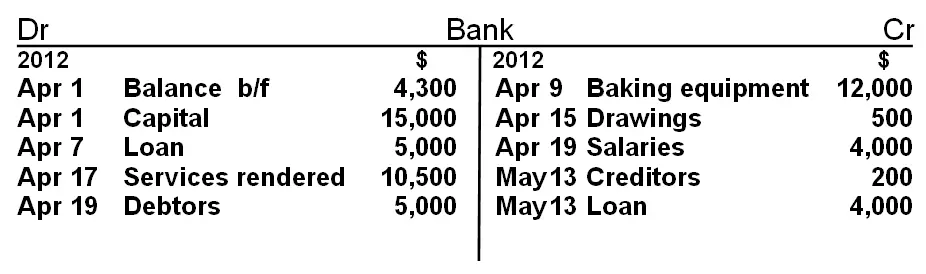In "Balance b/f" the "b/f" stands for "brought forward."

Sometimes this is written as "b/d," which stands for "brought down."

At the end of each accounting period (month or year) a brief calculation is done to work out the closing balance of the account.

How do we work out this closing balance?

It's done like this:

## How to Balance a T-Account

### 1. Quickly look over the account to find the side which has the bigger total.It should be fairly apparent in this example that the debit side is the bigger side.

### 2. Now add up the total of all the individual entries on this side and put it as a total below all the other amounts on this side.### 3. Put the same total on the other side below all the entries.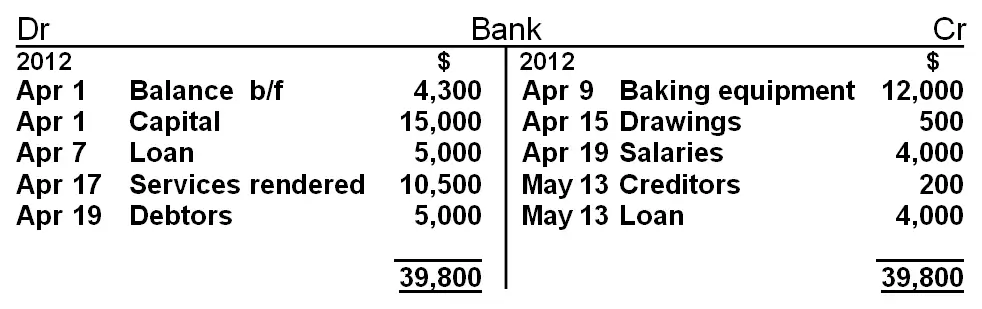### 4. Add up all the individual amounts on the smaller side.

This comes to \$20,700 in this example.

### 5. Work out the difference between this amount and the total inserted at the bottom.

\$39,800 – \$20,700 = \$19,100

### 6. Put this amount on the smaller side just above the total and describe it as "Balance c/f" or "Balance c/d," together with the date.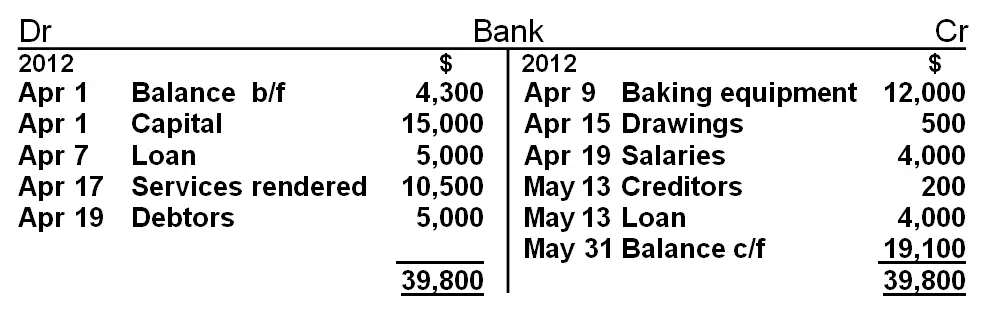This will ensure that the smaller side also adds up to the total.

### 7. Take this same amount (\$19,100) and insert it on the opposite side below the total, and describe this as "Balance b/f" or "Balance b/d".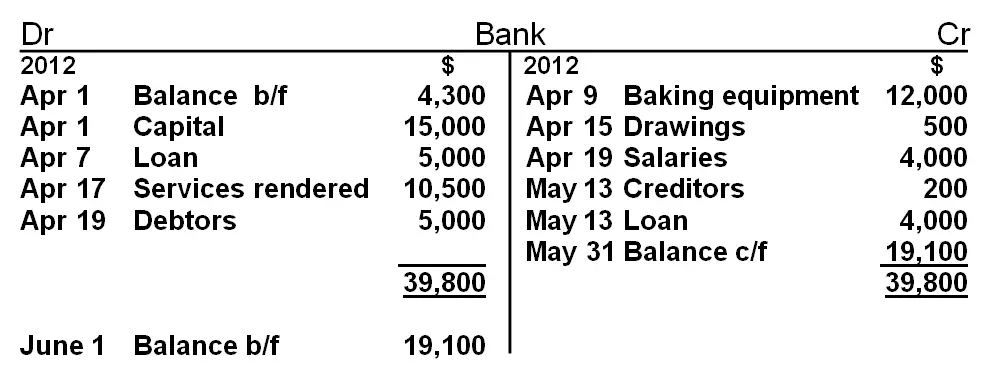### Balance B/F vs Balance C/F

The Balance b/f shown above is the actual closing balance of the bank account (a debit balance).

Balance c/f is just an entry used in calculating that the closing balance is \$19,100 on the debit side.

The "Balance b/f" indicates that the debit side is greater than the credit side by \$19,100, and that we have \$19,100 in our bank account at the end of May (the closing balance of the account).

Indeed, one could merely have taken the total of the debit side (\$39,800) and subtracted the total of the credit side (\$20,700) from this. We would arrive at the same answer: the bank account has a balance of \$19,100 on the debit side.

However, the steps taken above represent the system that is used in accounting to work out and show the closing balance, and thus should be learned and practiced.

So, we have our opening balance (debit) of \$4,300 and our closing balance (debit) of \$19,100. Both these balances can be determined by a quick examination of the T-account.

## Balancing a T-Account Practice Question

Let’s try another account from the sample business we've been using throughout our lessons, George's Catering – the "loan" T-account.

There were two transactions and journal entries involving the loan account: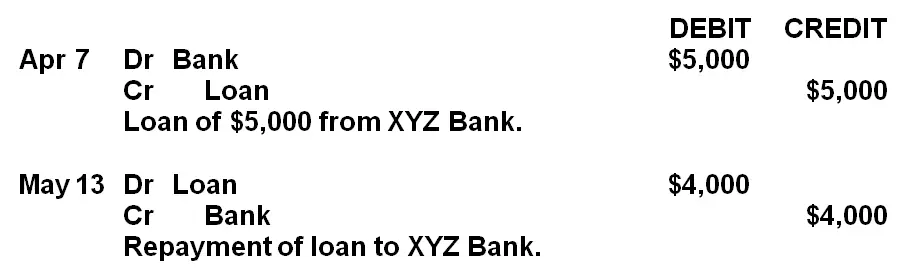So, what would the T-account look like?

Before going any further, take out a piece of paper and try construct the loan T-account using the journal entries above. When you're done, scroll down just below and compare your answers.

### Solution: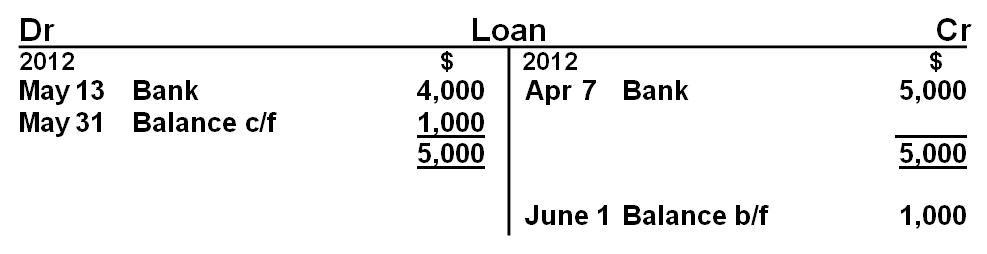If we were looking at this account for the first time, we would be able to see that \$5,000 was loaned on the 7th of April (a credit to the loan, meaning more of a liability), then \$4,000 was repaid on the 13th of May (a debit, meaning less of the liability), leaving us with an outstanding credit balance of \$1,000.

Remember, we can easily cross-reference between two accounts because of the contra account being used as the description of the transaction.

For example, in the "loan" account, "bank" is used as the description for the credit on the 7th of April.

And if you look in the "bank" account above, "loan" is inserted on the debit side of the T-account on the same date. We thus have an easy cross-reference.

## T-Accounts with Single Entries

With an account with just a single entry, we do the following to show the closing balance: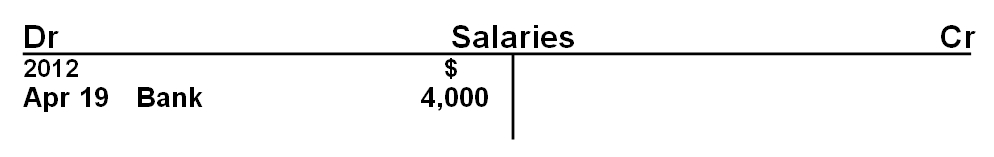We do not make any further entries to work out the closing balance – the \$4,000 balance is self-evident from the single entry.

## T-Account Codes (Folio Numbers)

Remember, each account has its own code or number (called a folio number), and this would normally be inserted next to the account name.

So the final prepared T-account would look like this: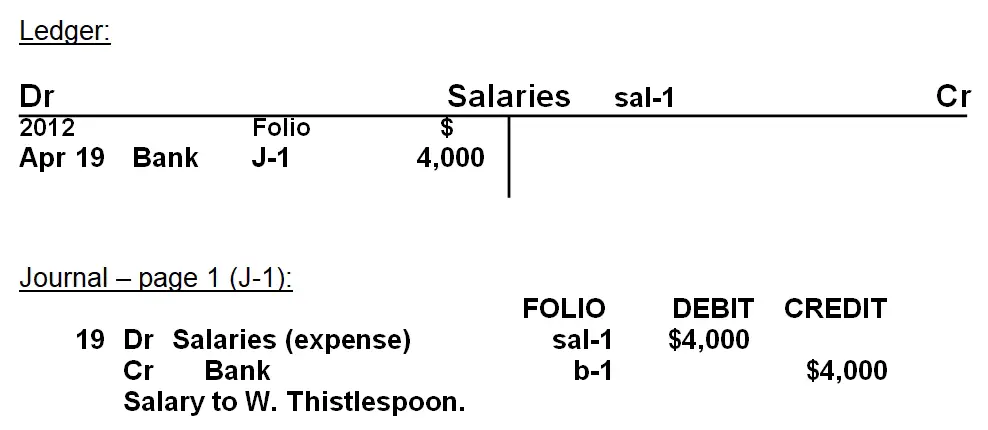"Sal-1" is the individual code for the account "salaries" and would also be referred to in the journal entries relating to salaries. "J-1" is the code for "journal page 1". The folio number or code thus helps with tracing information from the journal entry to the individual T-accounts, or from the ledger (T-accounts) back to the journal entries.

Hope that balancing T-accounts is a bit easier now!

If you're feeling good about how to balance a T-account, then go ahead and continue onto the next lesson, where we're going to learn more about how we post journals (meaning transfer the information from the journal entries into the T-accounts).

Stay up to date with ABfS!

Previous lesson: T Accounts and Ledgers
Next lesson: Posting Journal Entries to the Ledger

### Questions Relating to This Lesson

Click below to see questions and exercises on this same topic from other visitors to this page... (if there is no published solution to the question/exercise, then try and solve it yourself)

Ledgers
Q: What type of ledgers are there? A: 3 ledgers: - General Ledger - Debtor's Ledger - Creditor's Ledger

Balance C/F & Balance B/F Stand For...?
Question: Q: Please explain... What do Balance c/f & Balance b/f stand for? Answer: This is such a great question. So many accounting students see …

What is b/d and c/f?
Q: I want to ask - what is b/d and c/f? A: Good question Jibran. And great picture by the way! :) The term "b/d" stands for "brought down." …